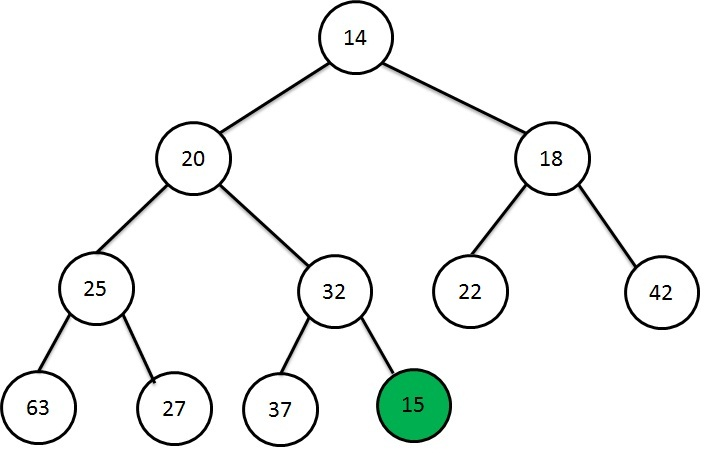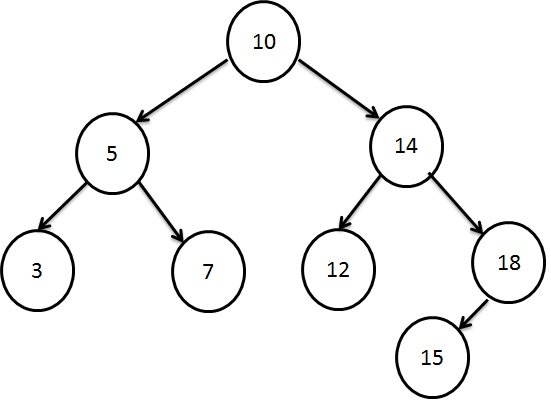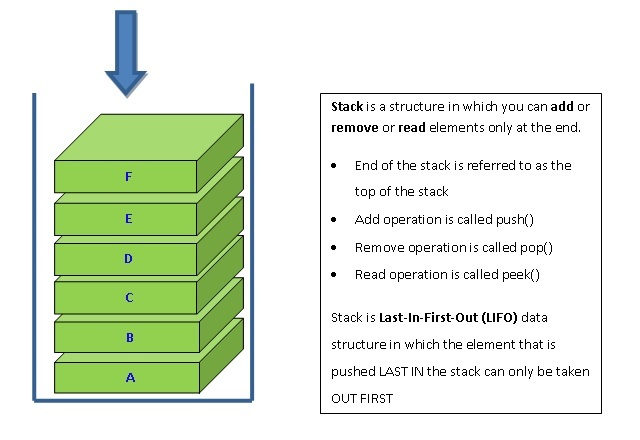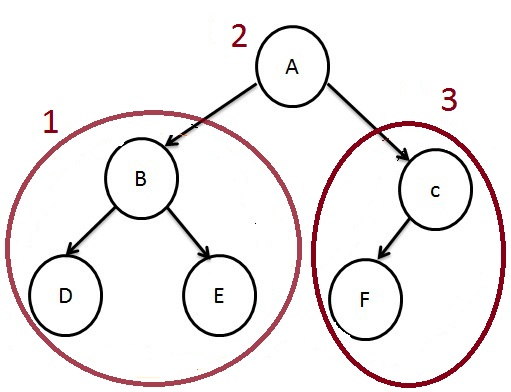# Graph - Degree, Indegree and Outdegree

### <<Previous Next >>

In this page, we will learn about quantifying the size or complexity of a graph.

## Quantifying the Graph

### Degree of a Vertex

Degree of vertex is the number of lines associated with a vertex. For example, let us consider the above graph. Degree of a vertex A is 1. Degree of a vertex B is 4. Degree of a vertex C is 2.

### Indegree of a Vertex

It is the number of arcs entering the vertex. For example, let us consider the above graph. Indegree of vertex B is 1.

### Outdegree of Vertex

It is the number of arcs leaving the vertex. For example, let us consider the above graph. Outdegree of vertex B is 3.

# Graph - Degree, Indegree and Outdegree

### <<Previous Next >>

In this page, we will learn about quantifying the size or complexity of a graph.

## Quantifying the Graph

### Degree of a Vertex

Degree of vertex is the number of lines associated with a vertex. For example, let us consider the above graph. Degree of a vertex A is 1. Degree of a vertex B is 4. Degree of a vertex C is 2.

### Indegree of a Vertex

It is the number of arcs entering the vertex. For example, let us consider the above graph. Indegree of vertex B is 1.

### Outdegree of Vertex

It is the number of arcs leaving the vertex. For example, let us consider the above graph. Outdegree of vertex B is 3.

### << Previous Next >>Heap Data StructureSearching using Binary Search TreeStacking the DataHow to traverse a Tree?